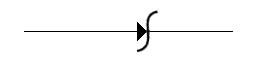# Electronics and Communication Engineering - Electronic Devices and Circuits

6.

Figure represents aA. Diode rectifier B. Schottky diode C. Varistor D. None of the above

Explanation:

No answer description available for this question. Let us discuss.

7.

The output, V-I characteristics of an Enhancement type MOSFET has

 A. only an ohmic region B. only a saturation region C. an ohmic region at low voltage value followed by a saturation region at higher voltages D. an ohmic region at large voltage values preceded by a saturation region at lower voltages

Explanation:

No answer description available for this question. Let us discuss.

8.

Piezoelectric quartz crystal resonators find application where

 A. signal amplification is required B. rectification of the signal is required C. signal frequency control is required D. modulation of signal is required

Explanation:

No answer description available for this question. Let us discuss.

9.

The forbidden energy gap between the valence band and conduction band will be least in case of

 A. metals B. semiconductors C. insulators D. all of the above

Explanation:

No answer description available for this question. Let us discuss.

10.

If too large current passes through the diode

 A. all electrons will leave B. all holes will freeze C. excessive heat may damage the diode D. diode will emit light